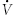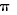# Useful equations and conversion factors

## Temperature conversions

Fahrenheit (°F) to Celsius (°C):

## Stack diameter

Where:

d = 2 √ A/π

d = diameter in m
π= 3.1416 (approximately)
A = cross-sectional area in m2

## Exit velocity of stack using a known volumetric flow rate

Where:= exit velocity in m/s= volumetric flowrate in m3/s
A = stack area in m2

## Calculation of stack gas flow rate for a cylindrical stack

Where:= volumetric flowrate in m3/s= exit velocity in m/s= 3.1416 (approximately)
d= internal stack diameter in m

## Determination of the stack gas flow rate, dry basis

Where:Dry= dry volumetric flowrate in m3/s= volumetric flow rate in m3/s (include water vapour)Water vapour= fraction of water vapour in stack effluent

## General conversion factors from Imperial to Metric units

General conversion factors from Imperial to Metric units
Convert from: To: Multiply by:
pounds (lb) kilograms (kg) 0.453592
ton (short ton) tonnes 0.907185
foot (ft) metres (m) 0.304804
gallon (U.S. gal) litres (L) 3.785412
cubic foot (ft3) cubic metres (m3) 0.0283168

## Power and energy conversions from Imperial to Metric Units

Power and energy conversions from Imperial to Metric units
Convert from: To: Multiply by:
million BTUs/hour kilowatts (kW) 293.1
million BTUs/hour Horsepower (Hp) 393.0
million BTUs kilojoules (kJ) 1.055 million
million BTUs Gigajoules (GJ) 1.055

## Emission factor conversions: Imperial to Metric Units

Emission factor conversions: Imperial to Metric units
Convert from: To: Multiply by:
lb/1 000 gal kg/1 000 L 0.1198
lb/ton kg/tonne 0.500
lb/million BTUs kg/million BTUs 0.454
lb/1 000 ton kg/1 000 tonnes 0.500
lb/million ft3 kg/million m3 16.018